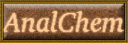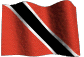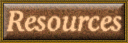HOME Email

# Ohm's Law

 Volts = √ watts x ohms Watts = volts x amperes Volts = watts ÷ amperes Watts = amperes2 x ohms Volts = amperes x ohms Watts = volts2 ÷ ohms Ohms = volts ÷ amperes Amperes = volts ÷ ohms Ohms = volts2 ÷ watts Amperes = watts ÷ volts Ohms = watts ÷ amperes2 Amperes = √ watts ÷ ohms